Select Page

# Differential Equations 12 Science Maths CBSE Solutions for MCQ in English

Differential Equations 12 Science Maths CBSE Solutions for MCQ in English to enable students to get Solutions in a narrative video format for the specific question.

Expert Teacher provides Differential Equations 12 Science Maths CBSE Solutions for MCQ through Video Solutions in English language. This video solution will be useful for students to understand how to write an answer in exam in order to score more marks. This teacher uses a narrative style for a question from Differential Equations not only to explain the proper method of answering question, but deriving right answer too.

Please find the question below and view the Solution in a narrative video format.

Question:

Solution Video in English:

You can select video Solutions from other languages also. Please check Solutions in ( Hindi )

## Similar Questions from CBSE, 12th Science, Maths, Differential Equations

Question 1 : Find the particular solution of the differential equation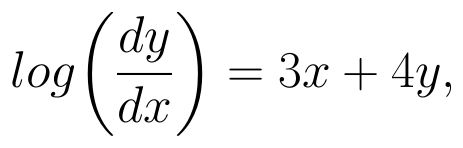given that y = 0, when x = 0. (View Answer Video)

Question 2 : Solve the differential equation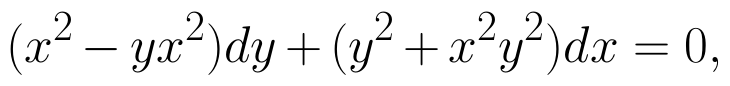given that y = 1 when x = 1. (View Answer Video)

Question 3 : Find the sum of the order and the degree of the following differential equation: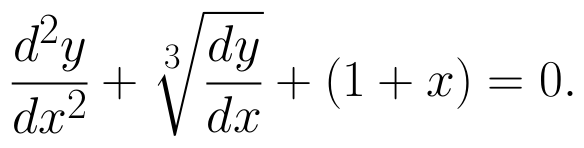(View Answer Video)

Question 4 : Find the particular solution of the differential equation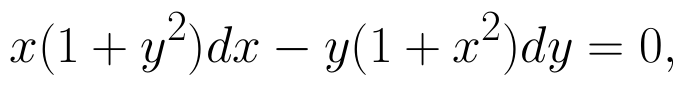given that y = 1 when x = 0.  (View Answer Video)

Question 5 :  Find the particular solution of the differential equation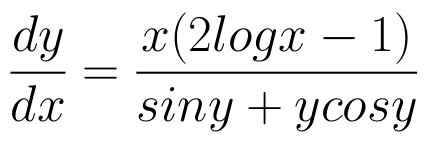given that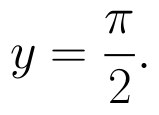When x =1.  (View Answer Video)

### Linear Programming

Question 1 : The objective function is maximum or minimum, which lies on the boundary of the feasible region. (View Answer Video)

### Continuity and Differentiability

Question 1 : Differentiate w.r.t.x the function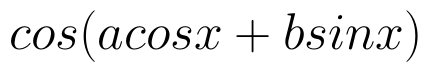, for some constant a and b. (View Answer Video)

Question 2 : Differentiate the function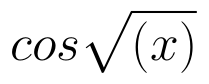with respect to x. (View Answer Video)

Question 3 : Differentiate the function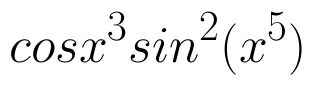with respect to x. (View Answer Video)

Question 4 : Differentiate the function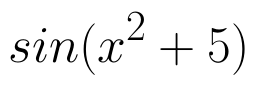with respect to x. (View Answer Video)

Question 5 : Differentiate the function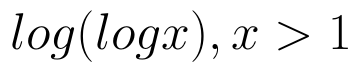with respect to x. (View Answer Video)

### Three Dimensional Geometry

Question 1 : Write the distance between the parallel planes 2x - y + 3z = 4 and 2x - y + 3z = 18. (View Answer Video)

Question 2 : Find the distance of point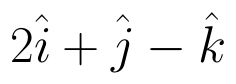from the  plane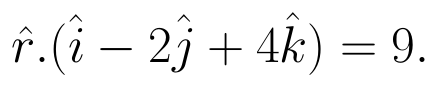(View Answer Video)

Question 3 : If a line marks angles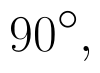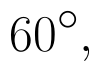and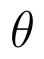with x, y and z-axis respectively, whereis acute, then find. (View Answer Video)

Question 4 : If lines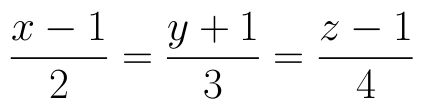and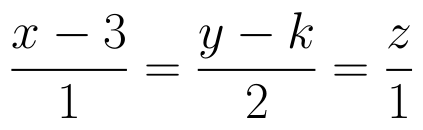intersect, then find the value of k and hence find the equation of plane containing these lines. (View Answer Video)

Question 5 : Find the vector equation of the plane which is at a distance of 5 units from the origin and normal to the plane is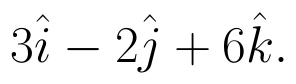(View Answer Video)